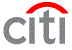# Placement Papers - CiticorpCiticorp Placement Paper Pattern (Aptitude/Technical)
Posted by :
Nikhil Tambi
(22)
PAPER : Citicorp Placement Paper Pattern (Aptitude/Technical)
The Written Test consists of three sections. The first section is an aptitude section. The second section is a 10 data interpretation questions section. For this section you follow the GRE book. In these questions some graphs will be there and the related questions will be asked...so you prepare from gre book. The third section is a Number series with 20 Questions to be done in 7 minutes.

The Forth section is a Figure series with 20 Questions to be done in 14 minutes. The last section is a Essay. In the last section there was passage. You should conclude that one in the specified 10 min. It would be better to prepare a computer related topics like client server, internet etc..

SAMPLE PAPER Aptitude:-
1. For the first qustion in the paper answer is (30).
2. Five trays cost is 0.35 each and dozen----- (ans:Rs13.75)
3. In a journey of 15 miles two third distance was travelled with 40 mph and remaining with 60 mph.How muvh time the journey takes...(ans:20 min)
4. A man walks from 9.15 to 5.15 from monday to friday and 9.00 to 12.00 on saturday.Each day 0.45 min lunch.How much time he walks in a week...(ans: 39hrs15min).

5. 12 revolutions takes 1/8 th second time.In 20 seconds how many revolutions...(ans:1920)
6. In 60 reems of paper 40 reems were utilised then what percent will remain...(ans:33.33%)
7. A started at 9.00 am with 6 mph and B started at 9.30 am with 8mph in the same direction. At what time they will meet... (ans:11.00am)
8. In a storage stall of 5x3x2inch.How many blanks of size 2x1x1inch can be stored..(ans:15)
9. In a company 3/5 of people know shorthand 1/4th know typing and 1/5 know both. What fraction of people do not know both.. (ans:35%)
10. A man how many bikes of Rs 14000 can buy by selling 2100 bikes of Rs500 of each (ans:75)
11. A company requires 11,500 strength.present employees are 200 women , men and 6500 unmarried To reach the target how many women required to maintain the same ratio (ans : 300)

12. What is the time required to punch 1500 cards of 50 column each at the rate of 10,000 punches per hour (ans:7hr 30min)
13. In a group of five persons A,B,C,D,and E
a)A and C are intelligent in English and Reasoning.
b)B and C are intelligent in English and General Awareness.
c)E and D are intelligent in Arithmatic and Inteview.
d)E is intelligent in Interview,reasoning and Arithe matic.
e)B and D are intelligent in Arithematic and General Awareness.

1) Who is intelligent in English,arithematic,and general awareness (ANSWER:B)
2)Who is intelligent in english and reasoning but not in general awareness (ans:A)
3)Who is intelligent in Arithematic,General Awareness and Interview (ans:D)
4)Who is intelligent in English General Awareness and Reasoning (ans:C)
5)Who is intelligent in Arithematic,Reasoning and Interview(ans:E)

14. Five persons A,B,C,D and E were travelling in a car.They were two ladies in the group. Of them who knew the car driving one was a lady.A is brother of D.B wifeof D,drve at the begining. E drive at the end.

1)Which of the following is a pair of brothers.(ans:A,D)
2)Who was the other lady in the group.(ans:C)
3)In the case of which the following pairs,no relationship can be established with one else in the group (ans:C,E)
4)How was E related to A.(ans:DATA INADEQUATE)

15. A man said to woman " your only brother\'s son is my wife\'s brother". How is the woman related to the man\'s wife...(ans:AUNT)

Technical:-
1. The following variable is available in file1.c
static int average_float;
all the functions in the file1.c can access the variable

2. extern int x;

3. Another Problem with
# define TRUE 0
some code
while(TRUE)
{
some code

}
This won\'t go into the loop as TRUE is defined as 0

4. A question in structures where the memebers are dd,mm,yy.
mm:dd:yy
09:07:97

5. A question with argc and argv .
Input will be
c:TEMP.EXE Ramco Systems India

6.
main()
{
int x=10,y=15;
x=x++;
y=++y;
printf("%d %d
",x,y);
}
find the output?

7.
int x;
main()
{
int x=0;
{
int x=10;
x++;
change_value(x);
x++;
Modify_value();
printf("First output: %d
",x);
}
x++;
change_value(x);
printf("Second Output : %d
",x);
Modify_value();
printf("Third Output : %d
",x);
}

Modify_value()
{
return (x+=10);
}

change_value()
{
return(x+=1);
}
find the output?

8.
{
int x=20,y=35;
x = y++ + x++;
y = ++y + ++x;
printf("%d %d
",x,y);
}
find the output?

9.
main()
{
char *p1="Name";
char *p2;
p2=(char *)malloc(20);
while(*p2++=*p1++);
printf("%s
",p2);
}
find the output?

10.
main()
{
int x=5;
printf("%d %d %d
",x,x<<2,x>>2);
}
find the output?

11.
#define swap1(a,b) a=a+b;b=a-b;a=a-b;
main()
{
int x=5,y=10;
swap1(x,y);
printf("%d %d
",x,y);
swap2(x,y);
printf("%d %d
",x,y);
}

int swap2(int a,int b)
{
int temp;
temp=a;
b=a;
a=temp;
return;
}
find the output?

12.
main()
{
char *ptr = "Ramco Systems";
(*ptr)++;
printf("%s
",ptr);
ptr++;
printf("%s
",ptr);
}
find the output?

13.
#include<stdio.h>
main()
{
char s1[]="Ramco";
char s2[]="Systems";
s1=s2;
printf("%s",s1);
}
find the output?

14.
#include<stdio.h>
main()
{
char *p1;
char *p2;
p1=(char *) malloc(25);
p2=(char *) malloc(25);
strcpy(p1,"Ramco");
strcpy(p2,"Systems");
strcat(p1,p2);
printf("%s",p1);
}